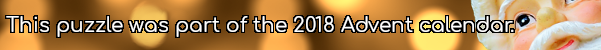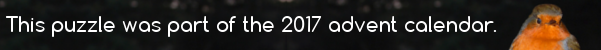mscroggs.co.uk
mscroggs.co.uksubscribe

# Puzzles

## 11 DecemberThis puzzle is inspired by a puzzle Woody showed me at MathsJam.
Today's number is the number $$n$$ such that $$\frac{216!\times215!\times214!\times...\times1!}{n!}$$ is a square number.

## Square and cube endings

Source: UKMT 2011 Senior Kangaroo
How many positive two-digit numbers are there whose square and cube both end in the same digit?

## 16 DecemberThere are 204 squares (of any size) in an 8×8 grid of squares. Today's number is the number of rectangles (of any size) in a 2×19 grid of squares

## 14 DecemberThere are 204 squares (of any size) in an 8×8 grid of squares. Today's number is the number of squares in a 13×13 grid of squares

## What's the star?

In the Christmas tree below, the rectangle, baubles, and the star at the top each contain a number. The square baubles contain square numbers; the triangle baubles contain triangle numbers; and the cube bauble contains a cube number.
The numbers in the rectangles (and the star) are equal to the sum of the numbers below them. For example, if the following numbers are filled in:
then you can deduce the following:
What is the number in the star at the top of this tree?

## Square pairs

Source: Maths Jam
Can you order the integers 1 to 16 so that every pair of adjacent numbers adds to a square number?
For which other numbers $$n$$ is it possible to order the integers 1 to $$n$$ in such a way?

## Square factorials

Source: Woody at Maths Jam
Multiply together the first 100 factorials:
$$1!\times2!\times3!\times...\times100!$$
Find a number, $$n$$, such that dividing this product by $$n!$$ produces a square number.

## Lots of ones

Is any of the numbers 11, 111, 1111, 11111, ... a square number?

## Archive

Show me a random puzzle
▼ show ▼